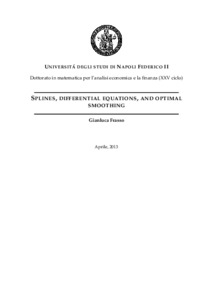Ricerca
Browse
Informazioni

Frasso, Gianluca (2013) Splines, differential equations and optimal smoothing. [Tesi di dottorato]Preview
Text
Splines, differential equations and optimal smoothing.pdf

[error in script] [error in script]
Item Type: Tesi di dottorato
Lingua: English
Title: Splines, differential equations and optimal smoothing
Creators:
CreatorsEmail
Frasso, Gianlucagianluca.frasso@unina.it
Date: 2 April 2013
Number of Pages: 166
Institution: Università degli Studi di Napoli Federico II
Department: Matematica e statistica
Scuola di dottorato: Scienze economiche e statistiche
Dottorato: Matematica per l'analisi economica e la finanza
Ciclo di dottorato: 25
Coordinatore del Corso di dottorato:
nomeemail
Cocozza, Rosarosa.cocozza@gmail.com
Tutor:
nomeemail
Siciliano, Robertaroberta@unina.it
Date: 2 April 2013
Number of Pages: 166
Uncontrolled Keywords: Differential equations, penalized smoothing splines, optimal smoothing
Settori scientifico-disciplinari del MIUR: Area 13 - Scienze economiche e statistiche > SECS-S/01 - Statistica
Area 13 - Scienze economiche e statistiche > SECS-S/06 - Metodi matematici dell'economia e delle scienze attuariali e finanziarie
Date Deposited: 10 Apr 2013 07:08
URI: http://www.fedoa.unina.it/id/eprint/9385
DOI: 10.6092/UNINA/FEDOA/9385## Abstract

In many scientific areas it is of primary interest to describe the dynamics of a system, that is, how it evolves over time and/or space. In the simple one-dimensional case the state of a system at any time can be represented by a function u(t) which' values track the evolution of a given phenomenon over time. It is also possible to consider phenomena evolving in time and space by using a function u(t,x) depending on two independent variables. Thus knowing t and/or x it is possible to evaluate the state of the system at at a given point in space and/or time. One way to obtain u(.) is taking measurements at different values of the independent variable(s) and fit the data in order to estimate a formula for u. This is the point of view exploited in statistical data analysis. Overparametric regression (smoothing) techniques are usually applied in this kind of studies. On the other hand it is clear that such a model would tell us how the system evolves but is not able to clarify why the system behaves as has been observed. Therefore we try to formulate mathematical models summarizing the understanding we seek. Often these models are dynamic equations that relate the state function to one or more of its derivatives w.r.t. the independent variable(s). Such equations are called differential equations (or abbreviated as DE). Differential equations are common analytic tools in physics and engineering. The first approach we have cited has the advantage to make the description of an observed phenomenon really flexible, being able to exploit all the information provided by the observed measurements. It becomes clear if we consider the applicability of overparametric smoothing techniques. These approaches, by the way, do not allow for a physical interpretation of the observed dynamics. On the other hand, the main advantage of a differential modeling point of view is to highlight the physical determinants of a given phenomenon but completely ignore what has been observed. The aim of this work is to present a flexible way to combine the statistical and the dynamic modeling points of view. To reach this goal we combine in a convenient way the flexible data description provided by a semi-parametric regression analysis and the physical interpretability of dynamics summarized by differential equations.